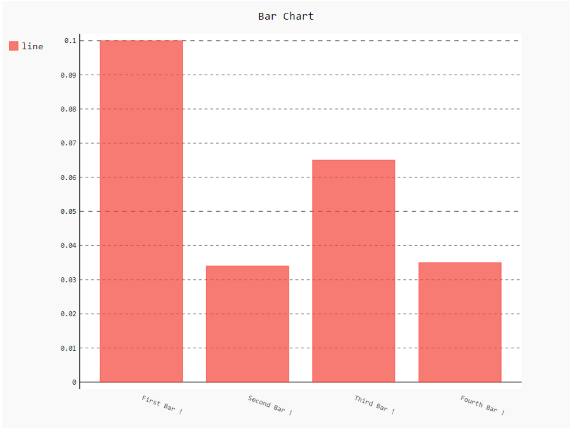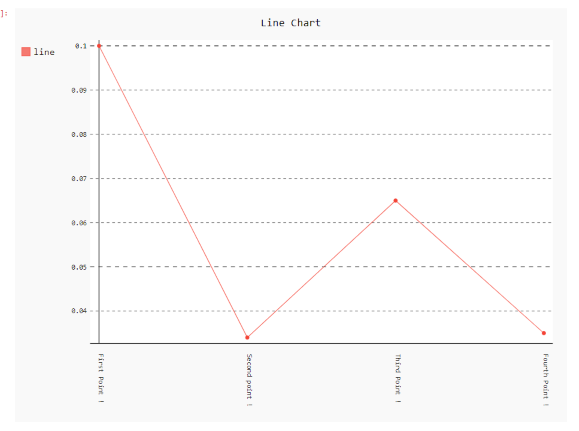Open In App

# How to rotate x labels using Pygal?

Prerequisites: Pygal

Pygal is a graphics and user interface library for Python that provides functionality commonly required in designing and science applications. While making a plot it is important for us to optimize its label, title, size. In this article, we will see how we can rotate the X-Label value of the plot window in the Pygal module. This can be done by passing the angle of rotation to x_label_rotation parameter.

Here are various ways to change the default plot x_label as per our requirement.

Approach:

• Import required module.
• Create a chart object.
• Pass x_label_rotation value in function.
• Label the graph.
• Display Graph.

Implementation of the concept discussed above is given below:

Example 1:

## Python3

 `# import library``import` `pygal``import` `numpy`` ` `# creating the chart object``chart ``=` `pygal.Bar(x_label_rotation``=``20``)`` ` `# Random data``chart.x_labels ``=` `[``    ``'First Bar !'``,``    ``'Second Bar !'``,``    ``'Third Bar !'``,``    ``'Fourth Bar !'``]``chart.add(``'line'``, [``0.1``, .``034``, .``065``, .``035``])`` ` `# naming the title``chart.title ``=` `'Bar Chart'`` ` `chart.render_to_png(``'img.png'``);`

Output:Example 2:

## Python3

 `# importing pygal``import` `pygal``import` `numpy`` ` `# creating the chart object``chart ``=` `pygal.Line(x_label_rotation``=``90``)`` ` `# Random data``chart.x_labels ``=` `[``    ``'First Point !'``,``    ``'Second point !'``,``    ``'Third Point !'``,``    ``'Fourth Point !'``]``chart.add(``'line'``, [``0.1``, .``034``, .``065``, .``035``])`` ` `# naming the title``chart.title ``=` `'Line Chart'`` ` `chart.render_to_png(``'img.png'``)`

Output: